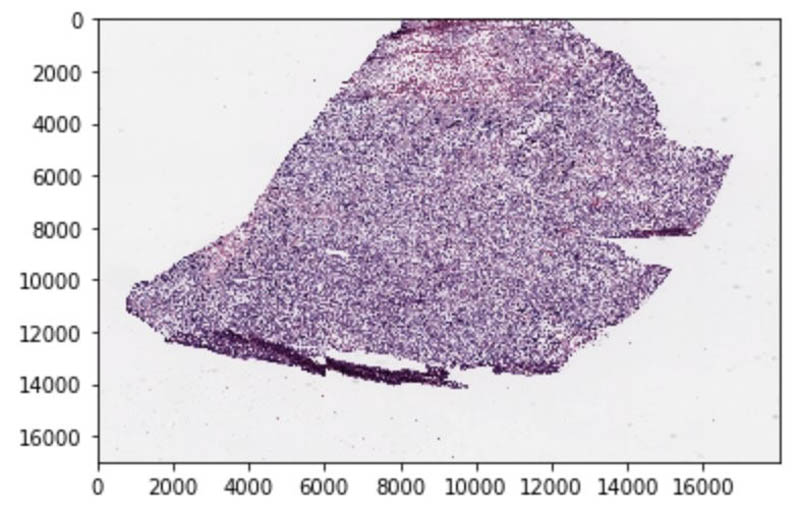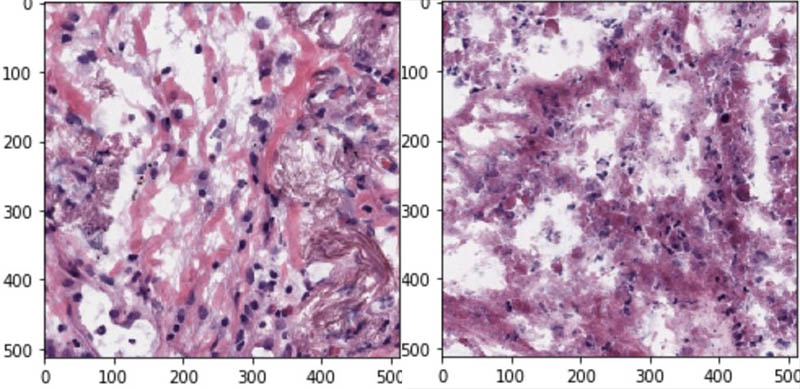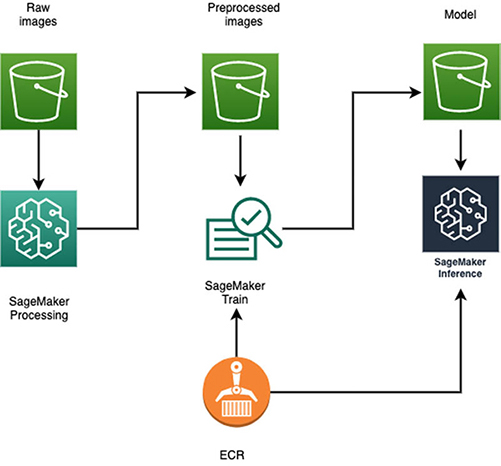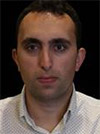Neural networks have proven effective at solving complex computer vision tasks such as object detection, image similarity, and classification. With the evolution of low-cost GPUs, the computational cost of building and deploying a neural network has drastically reduced. However, most techniques are designed to handle pixel resolutions commonly found in visual media. For example, typical resolution sizes are 544 and 416 pixels for YOLOv3, 300 and 512 pixels for SSD, and 224 pixels for VGG. Training a classifier over a dataset consisting of gigapixel images (10^9+ pixels) such as satellite or digital pathology images is computationally challenging. These images cannot be directly input into a neural network because each GPU is limited by available memory. This requires specific preprocessing techniques such as tiling to be able to process the original images in smaller chunks. Furthermore, due to the large size of these images, the overall training time tends to be high, often requiring several days or weeks without the use of proper scaling techniques such as distributed training.

In this post, we explain how to build a highly scalable machine learning (ML) pipeline to fulfill three objectives:

## Dataset

In this post, we use a dataset consisting of whole-slide digital pathology images obtained from The Cancer Genome Atlas (TCGA) to accurately and automatically classify them as LUAD (adenocarcinoma), LUSC (squamous cell carcinoma), or normal lung tissue, where LUAD and LUSC are the two most prevalent subtypes of lung cancer. The dataset is available for public use by NIH and NCI.

The raw high-resolution images are in SVS format. SVS files are used for archiving and analyzing Aperio microscope images. You can apply the techniques and tools used in this post to any ultra high-resolution image dataset, including satellite images.

The following is a sample image of a tissue slide. This single image contains over a quarter of a billion pixels, and occupies over 750 MB of memory. This image cannot be fed directly to a neural network in its original form, so we must tile the image into many smaller images.The following are samples of tiled images generated after preprocessing the preceding tissue slide image. These RGB 3-channel images are of size 512×512 and can be directly used as inputs to a neural network. Each of these tiled images is assigned the same label as the parent slide. Additionally, tiled images with more than 50% background are discarded.## Architecture overview

The following figure shows the overall end-to-end architecture, from the original raw images to inference. First, we use SageMaker Processing to tile, zoom, and sort the images into train and test splits, and then package them into the necessary number of shards for distributed SageMaker training. Second, a SageMaker training job loads the Docker container from Amazon Elastic Container Registry (Amazon ECR). The job uses Pipe mode to read the data from the prepared shards of images, trains the model, and stores the final model artifact in Amazon Simple Storage Service (Amazon S3). Finally, we deploy the trained model on a real-time inference endpoint that loads the appropriate Docker container (from Amazon ECR) and model (from Amazon S3) to process inference requests with low latency.## Data preprocessing using SageMaker Processing

The SVS slide images are preprocessed in three steps:

• Tiling images – The images are tiled by non-overlapping 512×512 pixel windows, and tiles containing over 50% background are discarded. The tiles are stored as JPEG images.
• Converting images to TFRecords – We use SageMaker Pipe mode to reduce our training time, which requires the data to be available in a proto-buffer format. TFRecord is a popular proto-buffer format used for training models with TensorFlow. We explain SageMaker Pipe mode and proto-buffer format in more detail in the following section.
• Sorting TFRecords – We sort the dataset into test, train, and validation cohorts for a three-way classifier (LUAD/LUSC/Normal). The TCGA dataset can have multiple slide images corresponding to a single patient. We need to make sure all the tiles generated from slides corresponding to the same patient occupy the same split to avoid data leakage. For the test set, we create per-slide TFRecord containing all the tiles from that slide so that we can evaluate the model in the way it will be used in deployment.

The following is the preprocessing code:

``````def generate_tf_records(base_folder, input_files, output_file, n_image, slide=None):
record_file = output_file

count = n_image
with tf.io.TFRecordWriter(record_file) as writer:
while count:
filename, label = random.choice(input_files)
if temp_img.shape != (512, 512, 3):
continue
count -= 1

image_string = np.float32(temp_img).tobytes()
slide_string = slide.encode('utf-8') if slide else None
tf_example = image_example(image_string, label, slide_string)
writer.write(tf_example.SerializeToString())
``````

We use SageMaker Processing for the preceding preprocessing steps, which allows us to run data preprocessing or postprocessing, feature engineering, data validation, and model evaluation workloads with SageMaker. Processing jobs accept data from Amazon S3 as input and store processed output data back into Amazon S3.

A benefit of using SageMaker Processing is the ease of distributing inputs across multiple compute instances. We can simply set` s3_data_distribution_type=ShardedByS3Key` parameter to divide data equally among all processing containers.

Importantly, the number of processing instances matches the number of GPUs we will use for distributed training with Horovod (i.e., 16). The reasoning becomes clearer when we introduce Horovod training.

The processing script is available on GitHub.

``````processor = Processor(image_uri=image_name,
role=get_execution_role(),
instance_count=16,               # run the job on 16 instances
base_job_name="processing-base", # should be unique name
instance_type="ml.m5.4xlarge",
volume_size_in_gb=1000)

processor.run(inputs=[ProcessingInput(
source=f's3://<bucket_name>/tcga-svs', # s3 input prefix
s3_data_type="S3Prefix",
s3_input_mode="File",
s3_data_distribution_type="ShardedByS3Key", # Split the data across instances
destination='/opt/ml/processing/input')], # local path on the container
outputs=[ProcessingOutput(
source="/opt/ml/processing/output", # local output path on the container
destination=f's3://<bucket_name>/tcga-svs-tfrecords/' # output s3 location
)],
arguments=['10000'], # number of tiled images per TF record for training dataset
wait=True,
logs=True)
``````

## Distributed model training using SageMaker Training

Taking ML models from conceptualization to production is typically complex and time-consuming. We have to manage large amounts of data to train the model, choose the best algorithm for training it, manage the compute capacity while training it, and then deploy the model into a production environment. SageMaker reduces this complexity by making it much easier to build and deploy ML models. It manages the underlying infrastructure to train your model at petabyte scale and deploy it to production.

After we preprocess the whole-slide images, we still have hundreds of gigabytes of data. Training on a single instance (GPU or CPU) would take several days or weeks to finish. To speed things up, we need to distribute the workload of training a model across multiple instances. For this post, we focus on distributed deep learning based on data parallelism using Horovod, a distributed training framework, and SageMaker Pipe mode.

### Horovod: A cross-platform distributed training framework

When training a model with a large amount of data, the data needs to distributed across multiple CPUs or GPUs on either a single instance or multiple instances. Deep learning frameworks provide their own methods to support distributed training. Horovod is a popular framework-agnostic toolkit for distributed deep learning. It utilizes an allreduce algorithm for fast distributed training (compared with a parameter server approach) and includes multiple optimization methods to make distributed training faster. For more examples of distributed training with Horovod on SageMaker, see Multi-GPU and distributed training using Horovod in Amazon SageMaker Pipe mode and Reducing training time with Apache MXNet and Horovod on Amazon SageMaker.

### SageMaker Pipe mode

You can provide input to SageMaker in either File mode or Pipe mode. In File mode, the input files are copied to the training instance. With Pipe mode, the dataset is streamed directly to your training instances. This means that the training jobs start sooner, compute and download can happen in parallel, and less disk space is required. Therefore, we recommend Pipe mode for large datasets.

SageMaker Pipe mode requires data to be in a protocol buffer format. Protocol buffers are language-neutral, platform-neutral, extensible mechanisms for serializing structured data. TFRecord is a popular proto-buffer format used for training models with TensorFlow. TFRecords are optimized for use with TensorFlow in multiple ways. First, they make it easy to combine multiple datasets and integrate seamlessly with the data import and preprocessing functionality provided by the library. Second, you can store sequence data—for instance, a time series or word encodings—in a way that allows for very efficient and (from a coding perspective) convenient import of this type of data.

The following diagram illustrates data access with Pipe mode.#### Data sharding with SageMaker Pipe mode

You should keep in mind a few considerations when working with SageMaker Pipe mode and Horovod:

• The data that is streamed through each pipe is mutually exclusive of the other pipes. The number of pipes dictates the number of data shards that need to be created.
• Horovod wraps the training script for each compute instance. This means that data for each compute instance needs to be from a different shard.
• With the SageMaker Training parameter` S3DataDistributionType` set to `ShardedByS3Key`, we can share a pipe with more than one instance. The data is streamed in round-robin fashion across instances.

To illustrate this better, let’s say we use two instances (A and B) of type ml.p3.8xlarge. Each ml.p3.8xlarge instance has four GPUs. We create four pipes (P1, P2, P3, and P4) and set `S3DataDistributionType="ShardedByS3Key’`. As shown in the following table, each pipe equally distributes the data between two instances in a round-robin fashion. This is the core concept needed in setting up pipes with Horovod. Because Horovod wraps the training script for each GPU, we need to create as many pipes as there are GPUs per training instance.The following code shards the data in Amazon S3 for each pipe. Each shard should have a separate prefix in Amazon S3.

``````# Definite distributed training hyperparameters
train_instance_type="ml.p3.8xlarge'
train_instance_count = 4
gpus_per_host = 4
num_of_shards = gpus_per_host * train_instance_count

distributions = {'mpi': {
'enabled': True,
'processes_per_host': gpus_per_host
}
}
``````

``````# Sharding
client = boto3.client('s3')
result = client.list_objects(Bucket=s3://<bucket_name>, Prefix='tcga-svs-tfrecords/train/', Delimiter="/")

j = -1
for i in range(num_of_shards):
copy_source = {
'Bucket': s3://<bucket_name>,
'Key': result['Contents'][i]['Key']
}
print(result['Contents'][i]['Key'])
if i % gpus_per_host == 0:
j += 1
dest="tcga-svs-tfrecords/train_sharded/" + str(j) +'/' + result['Contents'][i]['Key'].split('/')
print(dest)
s3.meta.client.copy(copy_source, s3://<bucket_name>, dest)

# Define inputs to SageMaker estimator
svs_tf_sharded = f's3://<bucket_name>/tcga-svs-tfrecords'
shuffle_config = sagemaker.session.ShuffleConfig(234)
train_s3_uri_prefix = svs_tf_sharded
remote_inputs = {}

for idx in range(gpus_per_host):
train_s3_uri = f'{train_s3_uri_prefix}/train_sharded/{idx}/'
train_s3_input = s3_input(train_s3_uri, distribution ='ShardedByS3Key', shuffle_config=shuffle_config)
remote_inputs[f'train_{idx}'] = train_s3_input
remote_inputs['valid_{}'.format(idx)] = '{}/valid'.format(svs_tf_sharded)
remote_inputs['test'] = '{}/test'.format(svs_tf_sharded)
remote_inputs
``````

We use a SageMaker estimator to launch training on four instances of ml.p3.8xlarge. Each instance has four GPUs. Thus, there are a total of 16 GPUs. See the following code:

``````local_hyperparameters = {'epochs': 5, 'batch-size' : 16, 'num-train':160000, 'num-val':8192, 'num-test':8192}

estimator_dist = TensorFlow(base_job_name="svs-horovod-cloud-pipe",
entry_point="src/train.py",
role=role,
framework_version='2.1.0',
py_version='py3',
distribution=distributions,
volume_size=1024,
hyperparameters=local_hyperparameters,
output_path=f's3://<bucket_name>/output/',
instance_count=4,
instance_type=train_instance_type,
input_mode="Pipe")

estimator_dist.fit(remote_inputs, wait=True)
``````

The following code snippet of the training script shows how to orchestrate Horovod with TensorFlow for distributed training:

``````mpi = False
if 'sagemaker_mpi_enabled' in args.fw_params:
if args.fw_params['sagemaker_mpi_enabled']:
import horovod.keras as hvd
mpi = True
# Horovod: initialize Horovod.
hvd.init()
# Pin GPU to be used to process local rank (one GPU per process)
gpus = tf.config.experimental.list_physical_devices('GPU')
tf.config.experimental.set_visible_devices(gpus[hvd.local_rank()], 'GPU')
else:
hvd = None

callbacks = []
if mpi:
callbacks.append(hvd.callbacks.MetricAverageCallback())
if hvd.rank() == 0:
callbacks.append(ModelCheckpoint(args.output_dir + '/checkpoint-{epoch}.ckpt',
save_weights_only=True,
verbose=2))
else:
callbacks.append(ModelCheckpoint(args.output_dir + '/checkpoint-{epoch}.ckpt',
save_weights_only=True,
verbose=2))

train_dataset = train_input_fn(hvd, mpi)
valid_dataset = valid_input_fn(hvd, mpi)
test_dataset = test_input_fn()
model = model_def(args.learning_rate, mpi, hvd)
logging.info("Starting training")
size = 1
if mpi:
size = hvd.size()

model.fit(train_dataset,
steps_per_epoch=((args.num_train // args.batch_size) // size),
epochs=args.epochs,
validation_data=valid_dataset,
validation_steps=((args.num_val // args.batch_size) // size),
callbacks=callbacks,
verbose=2)
``````

Because Pipe mode streams the data to each of our instances, the training script cannot calculate the data size during training (which is needed to compute steps_per_epoch). The parameter is therefore provided manually as a hyperparameter to the TensorFlow estimator. Additionally, the number of data points must be specified so that it can be divided equally amongst the GPUs. An unequal division could lead to a Horovod deadlock, because the time taken by each GPU to complete the training process is no longer identical. To ensure that the data points are equally divided, we use the same of number of instances for preprocessing as the number of GPUs for training. In our example, this number is 16.

## Inference and deployment

After we train the model using SageMaker, we deploy it for inference on new images. To set up a persistent endpoint to get one prediction at a time, use SageMaker hosting services. To get predictions for an entire dataset, use SageMaker batch transform.

In this post, we deploy the trained model as a SageMaker endpoint. The following code deploys the model to an m4 instance, reads tiled image data from TFRecords, and generates a slide-level prediction:

``````# Generate predictor object from trained model
predictor = estimator_dist.deploy(initial_instance_count=1,
instance_type="ml.m4.xlarge")

# Tile-level prediction
raw_image_dataset = tf.data.TFRecordDataset(f'images/{local_file}') # read a TFrecord
parsed_image_dataset = raw_image_dataset.map(dataset_parser) # Parse TFrecord to JPEGs

pred_scores_list = []
for i, element in enumerate(parsed_image_dataset):
image = element.numpy()
label = element.numpy()
slide = element.numpy().decode()
if i == 0:
print(f"Making tile-level predictions for slide: {slide}...")

print(f"Querying endpoint for a prediction for tile {i+1}...")
pred_scores = predictor.predict(np.expand_dims(image, axis=0))['predictions']
pred_class = np.argmax(pred_scores)

if i > 0 and i % 10 == 0:
plt.figure()
plt.title(f'Tile {i} prediction: {pred_class}')
plt.imshow(image / 255)

pred_scores_list.append(pred_scores)
print("Done.")

# Slide-level prediction (average score over all tiles)
mean_pred_scores = np.mean(np.vstack(pred_scores_list), axis=0)
mean_pred_class = np.argmax(mean_pred_scores)
print(f"Slide-level prediction for {slide}:", mean_pred_class)
``````

The model is trained on individual tile images. During inference, the SageMaker endpoint provides classification scores for each tile. These scores are averaged out across all tiles to generate the slide-level score and prediction. The following diagram illustrates this workflow.A majority vote scheme would also be appropriate.

To perform inference on a large new batch of slide images, you can run a batch transform job for offline predictions on the dataset in Amazon S3 on multiple instances. Once the processed TFRecords are retrieved from Amazon S3, you can replicate the preceding steps to generate a slide-level classification for each of the new images.

## Conclusion

In this post, we introduced a scalable machine learning pipeline for ultra high-resolution images that uses SageMaker Processing, SageMaker Pipe mode, and Horovod. The pipeline simplifies the convoluted process of large-scale training of a classifier over a dataset consisting of images that approach the gigapixel scale. With SageMaker and Horovod, we eased the process by distributing inputs across multiple compute instances, which reduces training time. We also provided a simple but effective strategy to aggregate tile-level predictions to produce slide-level inference.

For more information about SageMaker, see Build, train, and deploy a machine learning model with Amazon SageMaker. For the complete example to run on SageMaker, in which Pipe mode and Horovod are applied together, see the GitHub repo.

### References

1. Nicolas Coudray, Paolo Santiago Ocampo, Theodore Sakellaropoulos, Navneet Narula, Matija Snuderl, David Fenyö, Andre L. Moreira, Narges Razavian, Aristotelis Tsirigos. “Classification and mutation prediction from non–small cell lung cancer histopathology images using deep learning”. Nature Medicine, 2018; DOI: 10.1038/s41591-018-0177-5
2. https://github.com/ncoudray/DeepPATH/tree/master/DeepPATH_codeKaran Sindwani is a Data Scientist at Amazon Machine Learning Solutions where he builds and deploys deep learning models. He specializes in the area of computer vision. In his spare time, he enjoys hiking.Vinay Hanumaiah is a Deep Learning Architect at Amazon ML Solutions Lab, where he helps customers build AI and ML solutions to accelerate their business challenges. Prior to this, he contributed to the launch of AWS DeepLens and Amazon Personalize. In his spare time, he enjoys time with his family and is an avid rock climber.Ryan Brand is a Data Scientist in the Amazon Machine Learning Solutions Lab. He has specific experience in applying machine learning to problems in healthcare and the life sciences, and in his free time he enjoys reading history and science fiction.Tatsuya Arai, Ph.D. is a biomedical engineer turned deep learning data scientist on the Amazon Machine Learning Solutions Lab team. He believes in the true democratization of AI and that the power of AI shouldn’t be exclusive to computer scientists or mathematicians.